# Campus 101

6864 Registered Allowed team size: 1

This campaign is over.

hackathon
Online
starts on:
Dec 06, 2020, 06:24 PM
ends on:
Dec 06, 2020, 06:25 PM

## Algo - Milestone 1

#### Prerequisites

• Before you get started with algorithms, it is advisable that you have some basic level of knowledge in programming languages such as C, C++, Python. These are universal languages.
• You must attempt the data structures section provided in our program. It will help you to understand this section on algorithms better.

Note: It is important that you read through all the resource materials to be able to solve the multiple-choice questions and programming questions in your practice tests and the final test.

#### Introduction

Let’s assume that you want to prepare an omelette. To prepare this, you are required to perform the following steps: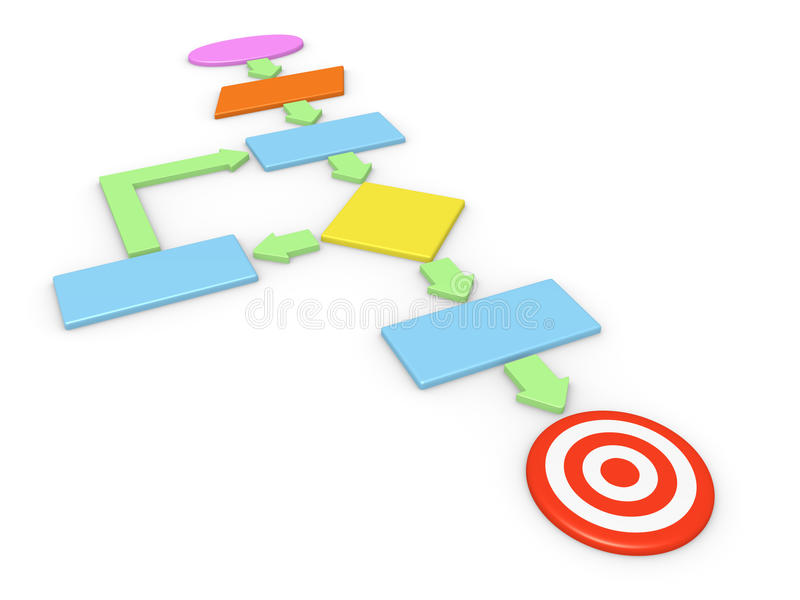1. Take a frying pan.
1. Do you have oil?
1. If yes, put it in the pan.
2. If no, do you want to buy oil?
1. If yes, then purchase it.
2. If no, do not do this task.
3. Turn the stove, etc…

This is a step-by-step procedure to solve a problem. Similarly, an algorithm represents step-by-step instructions that are provided to solve a given problem.

In computer science, there are multiple algorithms that are available to solve a problem. In the following milestones, you will learn the basic algorithms that will help you improve your programming skills and solve questions efficiently.

#### Sorting algorithms

Sorting is an algorithm that allows you to arrange the elements of a list in a certain order (either ascending or descending). Sorting can significantly reduce the complexity of a problem.

Classification of sorting

• Internal sort: A sorting algorithm that uses the main memory exclusively during the sorting process.
• External sort: A sorting algorithm that uses external memory such as tape or disk during the sorting process.

There are various types of external sorting algorithms. Some of these are as follows:

Bubble sort

It is also known as Sinking sort.

1. Each pair of adjacent items of a list are first compared and then swapped.
2. If adjacent elements are in an incorrect order then this process (step 1) continues until a fully sorted list is obtained.
3. With each pass of an element, the list places the element with the largest value in the correct position in the list.

The only advantage of this algorithm is that it can detect if the input list is already a sorted list or not.

The following table represents the first pass of a bubble sort for a list that contains 10, 4, 43, 5, 57, 91, 45, 9, 7. The shaded boxes represent that they are being compared to check the values for sorting.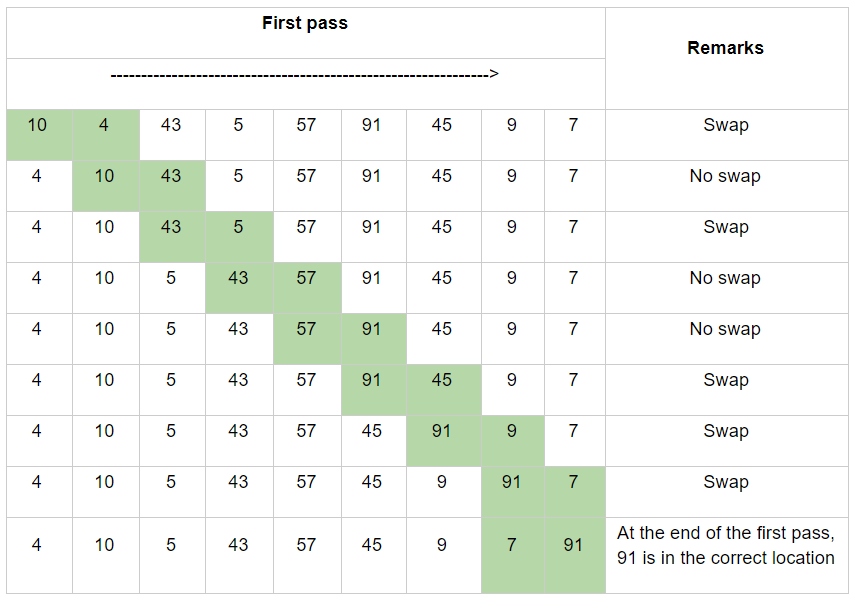Generally, if there are n-1 items to sort, then there are n-2 pairs. Since each pass places the next largest value in its correct location, the total number of passes necessary is n-1. After completing n-1 passes, the smallest item will be in the correct position with no further processing requirements.

You can implement the sorting for the provided list in the following way: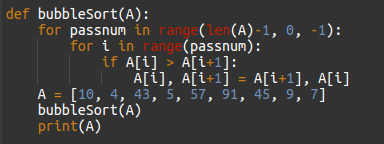If you are having difficulty in understanding this concept, click on this. It explains the same in an animated form.

Watch this fun dance video to understand bubble sort, Bubble sort dance.

Selection sort

In selection sort, only one exchange occurs for every pass through the list.

1. Find the largest value in the list.
2. Swap it with the value in the current position.
3. Repeat this process for all the elements until the entire array is sorted.

This sorting technique is used for sorting files with large values and small keys. This is because the selection is performed based on the keys and the swap operation is performed only when required.

The following table represents the selection sort on a list that contains 10, 4, 43, 5, 57, 91, 45, 9, 7. The shaded items represent that they are being compared to check the values for sorting.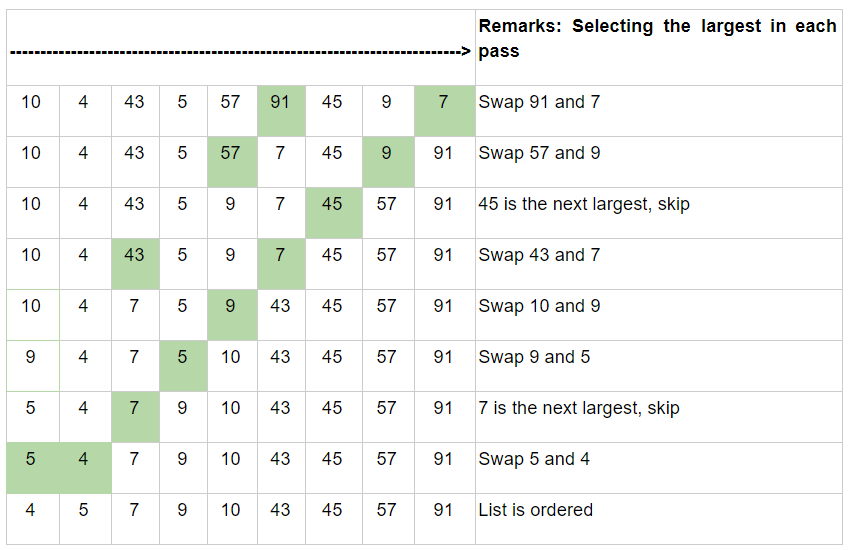Here, on each pass, the largest remaining item is selected and then placed in the correct location. The first pass places 91, the second pass places 57, the third pass places 45, and so on.

This can be implemented as follows: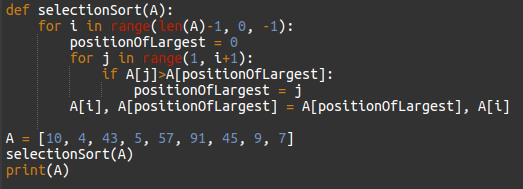Watch this fun dance video on selection sort.

Try to implement bubble sort on the same list but instead of selecting the largest remaining number, implement it by selecting the smallest remaining item.

Insertion sort

In this algorithm, each iteration removes an element from the input list and inserts it into the sorted list.

The following table represents the sixth pass. It contains a sorted sublist of 6 elements: 4, 5, 10, 43, 57, 91.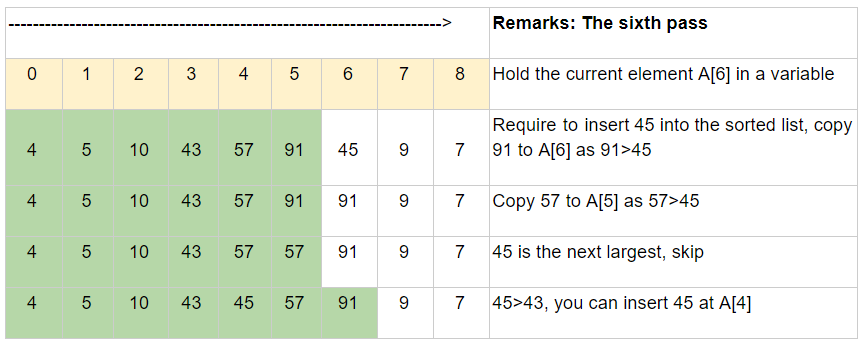Here, you want to insert 45 into the already sorted items. The first comparison with 91 makes 91 to shift to the right. As a result, 57 also must be shifted. When 43 is encountered, the shifting process stops and 45 is placed in the open position. Thus, providing a list of 7 sorted elements.

Watch this fun dance video on insertion sort.

Can you implement the algorithm on quick sort after watching this video? Try it out!

#### Searching algorithms

Searching is a process of finding an item with some specific properties from a collection of items. The item can be stored as records in a database, can be an array, text in files, nodes in a tree, vertices and edges in a graph, etc.

There are various types of searches that you can perform. Some of these are as follows:

• Unordered linear search
• Sorted/Ordered linear search
• Binary search
• Interpolation search
• Symbol tables and hashing
• String searching algorithms

Unordered linear search

Let’s assume that you are given an array where the order of the elements is unknown. In this, you are required to traverse the entire array and search the element. This can be performed as follows: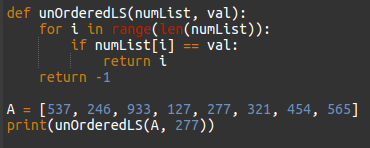Ordered linear search

If the elements of the array are already sorted, then you are not required to traverse the array. It can be implemented as follows: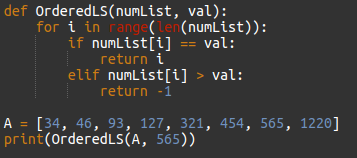Here, at any point, if the value at A[i] is greater than the element to be searched, then you return -1 without searching for the remaining elements in the array.

Binary search

Let’s say that you want to search a word in a dictionary. You directly go to some appropriate page (let’s say, the middle page) and start searching from that point. If the word you are searching for is on the same page then the search is complete. If the word is present before this middle page, you go back and search for the word. Similarly, if the word exists after the middle page, then you turn the following pages and check for the word.

Binary search works in the same way. The following code represents the iterative binary search algorithm: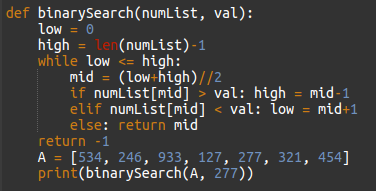Can you implement the recursive binary search algorithm?

Refer to this fun dance video on binary search.